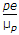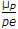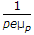# Electronics and Communication Engineering - Electronic Devices and Circuits

16.

When a diode is forward biased, the diode current is

 A. high B. low C. zero D. low or zero

Answer: Option A

Explanation:

No answer description available for this question. Let us discuss.

17.

In p type semiconductors the conduction due to holes ( = σp ) is (where e = charge on hole, μp is hole mobility and p is hole concentration)

 A.B.C. peμp D.Answer: Option C

Explanation:

No answer description available for this question. Let us discuss.

18.

At room temperature, the current in the, intrinsic semiconductor is due to

 A. holes B. electrons C. ions D. holes and electrons

Answer: Option D

Explanation:

No answer description available for this question. Let us discuss.

19.

Which of these is also called 'hot carrier diode'?

 A. PIN diode B. LED C. Photo diode D. Schottky diode

Answer: Option D

Explanation:

No answer description available for this question. Let us discuss.

20.

A d.c. power supply has an open circuit voltage of 100 V. When the full load current is drawn, the output drops to 80 V. The percentage voltage regulation is

 A. 97.25% B. 75% C. 50% D. 25%

Answer: Option D

Explanation:

No answer description available for this question. Let us discuss.

#### Current Affairs 2021

Interview Questions and Answers